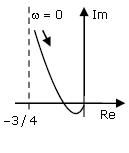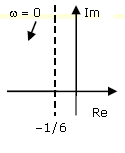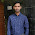## Wednesday, November 21, 2012

### Control Systems: Part2 Objective Questions

 The system represented by the input-output relationship y(t)= 5t-∞ x(τ)dτ, t>0 is
(a) Linear and casual
(b) Linear but not casual
(c) Casual but not linear
(d) Neither linear nor casual

 For the system 2/(s+1), the approximate time taken for a step response to reach 98% of its final value is
(a) 1s
(b) 2s
(c) 4s
(d) 8s

 Given the finite length input x[n] and the corresponding finite length output y[n] of an LTI system as shown below, the impulse response h[n] of the system is
(a) h[n]={1,0,0,1}
(b) h[n]={1,0,1}
(c) h[n]={1,1,1,1}
(d) h[n]={1,1,1}

 The frequency response of G(s)=1/[s(s+1)(s+2)] plotted in the complex G(jω) plane (for 0< ω<∞) is....Options A, B, C, D are given below
A.The system x=Ax+Bu with
A=
B=is

(a) Stable and controllable
(b) Stable but uncontrollable
(c) Unstable but controllable
(d) Unstable and uncontrollable

 The characteristic equation of a closed-loop system is a(s+1)(s+3)+k(s+2)=0,k>0. Which of the following statements is true?
(a) Its roots are always real
(b) It cannot have a breakaway point in the range -1<Re[s]<0
(c) Two of its roots tend to infinity along the asymptotes Re[s]=-1
(d) It may have complex roots in the right half plane

 The frequency response of a linear system G(jω) is provided in the tabular form below
 |G(jω)| 1.3 1.2 1.0 0.8 0.5 0.3 ∠G(jω) -130° -140° -150° -160° -180° -200°
(a)  6dB and 30°
(b)  6dB and -30°
(c)  -6dB and 30°
(d)  -6dB and -30°

 An openloop system represented by the transfer function G(s) = [(s+1)/(s+2)(s+3)] is
a) Stable and of the minimum phase type
b) Stable and of the non-minimum phase type
c) Unstable and of the minimum phase type
d) Stable and of the non-minimum phase type

 The open loop transfer function G(s) of a unity feedback control system is given as, G(s)=[ k(s+2/3) / s2(s+2) ] From the root locus, it can be inferred that when k tends to positive infinity.
(a)  Three roots with nearly equal real parts exist on the left half of the s-plane
(b)  One real root is found on the right half of the s-plane
(c)  The root loci cross the jω axis for a finite value of k; k not equal to 0
(d)  Three real roots are found on the right half of the s-plane

 The response h(t) of a linear time invariant system to an impulse δ(t), under initially relaxed condition is h(t) = e-t+e-2t. The response of this system for a unit step input u(t) is
(a)   u(t)+e-t+e-2t
(b)   (e-t+e-2t) u(t)
(c)   (1.5-e-t-0.5e-2t) u(t)
(d)   e-tδ(t)+e-2tu(t)

3:55 PM

#### 1 comment:

1.Question no. 8 the system is minimum phase system because all the poles and zero lie in the left half s-plane